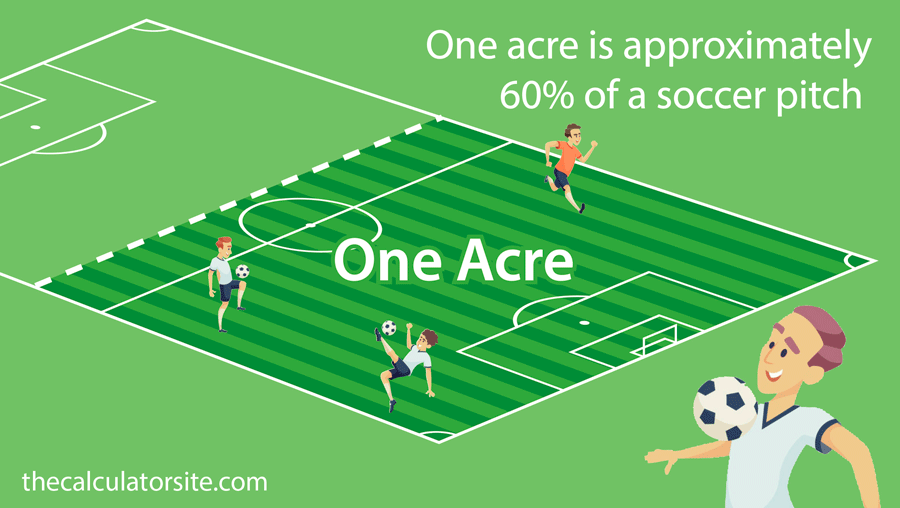# How many acres is a football field?

Well, other day, we were looking for a good answer to this question. But could not find a proper complete answer for this. So here it is. Let’s discuss about how many acres is a football field.Image source: Wiki

In America, the football field measures at 100 years that’s about 91.44m long between the goal line. There is also at end zone at each end which is 10 yards long. So all together the total length is 120 yards.

The width is uniform at 53 1/3 yards.

Final Calculation: The total area of a football field (Including end zones) – 57,600 square feet or 5351.22 square meters

One acre is 43,560 square feet or 4046.86 square meter. So,

### How many acres is a football field? – 1.32 acres

Now you might ask how long is an acre?

First of all an acre not necessarily have to be perfectly square or rectangle. In fact it can be round of triangle. Main thing to consider is the total area which have to be exactly 43,560 square feet. Or 4046.86 meters. If you want to know that in feet- an acre in feet is 660 feet by 66 feet.

### How many acres is a UK football fields?

In UK there are some flexibility in football field’s sizes. But overall the most common football field size is 110 yards of length with a width of 70 yards. So the size of football field of Uk is about 1.5 acres.Image source: thecalculatorsite.com

### FAQ

 Question: How many football fields is 5 acres? Answer: If the calculation is done correctly then it’s about 4.53 football fields. Question: How many acres is a soccer field? Answer: A standard soccer field is about .6 acres Question: How many square feet in an acre? Answer: Its 43560 square feet. Question: What are the dimensions of an acre? Answer: Acre is measured in area not length. So it can be any shapes. As long as the total area is 46,560 square feet then you call it an acre. Question: How many acres is a soccer field? Answer: A soccer field  is about 1.86 acres

Remember, football field sizes varies in different country. In America, almost all school, college and NFL fields follow this size. Also in Canada, they have very similar way of measuring their football fields except some minor changes. So the size of football fields is not universal you can call it more country or area specific.

[Total: 2   Average: 5/5]#### BestRevX

We will be happy to hear your thoughts